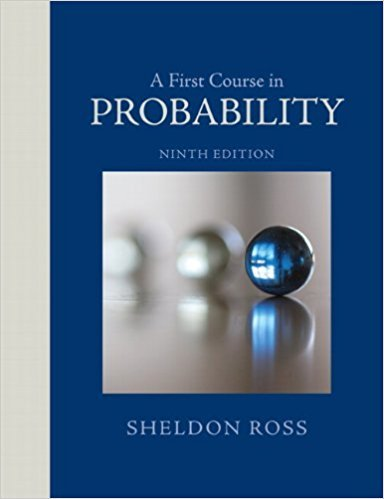×
Get Full Access to A First Course In Probability - 9 Edition - Chapter 4 - Problem 57p
Get Full Access to A First Course In Probability - 9 Edition - Chapter 4 - Problem 57p

×

# Suppose that the number of accidents occurring on aISBN: 9780321794772 63

## Solution for problem 57P Chapter 4

A First Course in Probability | 9th Edition

• Textbook Solutions
• 2901 Step-by-step solutions solved by professors and subject experts
• Get 24/7 help from StudySoup virtual teaching assistantsA First Course in Probability | 9th Edition

4 5 1 314 Reviews
17
2
Problem 57P

Problem 57P

Suppose that the number of accidents occurring on a highway each day is a Poisson random variable with parameter λ = 3.

(a) Find the probability that 3 or more accidents occur today,

(b) Repeat part (a) under the assumption that at least 1 accident occurs today.

Step-by-Step Solution:
Step 1 of 3

Solution 57P

Step1 of 3:

From the given problem we have a random variable ‘X’ it presents the number of accidents occurring on a highway each day. Here X follows poisson distribution with parameterHere our goal is:

a). We need to find the probability that 3 or more accidents occur today.

b). We need to find the probability that at least 1 accident occurs today.

Step2 of 3:

a).

Here the random variable X follows poisson distribution with parameterThen, the probability mass function of poisson distribution is:Consider,Therefore, The probability that 3 or more accidents occur today is 0.5767.

Step3 of 3:

b).

The probability that at least 1 accident occurs today is:Where,[from part (a)]Therefore, P(X1) = 0.9502.

Now,Therefore, The probability that at least 1 accident occurs today is 0.6069.

Step 2 of 3

Step 3 of 3

##### ISBN: 9780321794772

This full solution covers the following key subjects: accidents, today, parameter, day, Find. This expansive textbook survival guide covers 4 chapters, and 469 solutions. The full step-by-step solution to problem: 57P from chapter: 4 was answered by , our top Statistics solution expert on 08/11/17, 08:57AM. Since the solution to 57P from 4 chapter was answered, more than 781 students have viewed the full step-by-step answer. A First Course in Probability was written by and is associated to the ISBN: 9780321794772. This textbook survival guide was created for the textbook: A First Course in Probability , edition: 9. The answer to “Suppose that the number of accidents occurring on a highway each day is a Poisson random variable with parameter ? = 3.(a) Find the probability that 3 or more accidents occur today,________________(b) Repeat part (a) under the assumption that at least 1 accident occurs today.” is broken down into a number of easy to follow steps, and 45 words.

Unlock Textbook Solution